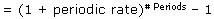# Annual Percentage Yield - APY

﻿
Annual Percentage Yield - APY
The effective annual rate of return taking into account the effect of compounding interest. APY is calculated by:The resultant percentage number assumes that funds will remain in the investment vehicle for a full 365 days.

The APY is similar in nature to the annual percentage rate. Its usefulness lies in its ability to standardize varying interest-rate agreements into an annualized percentage number.

For example, suppose you are considering whether to invest in a one-year zero-coupon bond that pays 6% upon maturity or a high-yield money market account that pays 0.5% per month with monthly compounding.

At first glance, the yields appear equal because 12 months multiplied by 0.5% equals 6%. However, when the effects of compounding are included by calculating the APY, we find that the second investment actually yields 6.17%, as 1.005^12-1 = 0.0617.

Investment dictionary. . 2012.